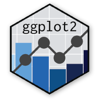Show Sidebar Hide Sidebar# geom_hex in ggplot2

How to make a hexagonal two-dimensional heatmap in ggplot2 using geom_hex. Examples of coloured and facetted graphs.

### New to Plotly?

Plotly's R library is free and open source!
You can set up Plotly to work in online or offline mode.
We also have a quick-reference cheatsheet (new!) to help you get started!

### Version Check

Version 4 of Plotly's R package is now available!
Check out this post for more information on breaking changes and new features available in this version.

library(plotly)
packageVersion('plotly')

##  '4.9.1'


### Basic 2d Heatmap

See also geom_bin2d for a similar geom with rectangular bins. Note: facetting is supported in geom_bin2d but not geom_hex.

library(plotly)

p <- ggplot(english_french, aes(x=engperc,y=frenperc)) +
geom_hex() +
labs(title = "Distribution of Canadian areas by English and French fluency",
x = "% fluent in English",
y = "% fluent in French",
fill = "# of census \nsubdivisions")
p <- ggplotly(p)

p


### Customized Colours

Let's flip the colour scheme so that lighter colours denote larger numbers than darker colours. We should also move to a logarithmic scale, since as it is, the very large value in the bottom right overshadows all other values.

library(plotly)

p <- ggplot(english_french, aes(x=engperc,y=frenperc)) +
geom_hex() +
labs(title = "Distribution of Canadian towns by English and French fluency",
x = "% fluent in English",
y = "% fluent in French",
fill = "# of census \nsubdivisions")
p <- ggplotly(p)

p


### Weighted Data

In the previous graphs, each observation represented a single census subdivision - this counted small towns of 500 people equally with cities like Montreal and Toronto. We can weight the data by the "total" column (i.e. total population) to make this a graph of population.

library(plotly)

p <- ggplot(english_french, aes(x=engperc, y=frenperc, weight=total)) +
geom_hex() +
labs(title = "Distribution of the Canadian population by English and French fluency",
x = "% fluent in English",
y = "% fluent in French",
fill = "population")
p <- ggplotly(p)

p


### Customized Appearance

We can modify the graph's appearance - for example, if the grey background makes it difficult to make out the paler shades of blue, we can change the theme to one with a white background. Included also is a way to change the font. You can find a list here of all the theme elements that you can modify.

library(plotly)

p <- ggplot(english_french, aes(x=engperc,y=frenperc, weight=total)) +
geom_hex(bins = 20) +